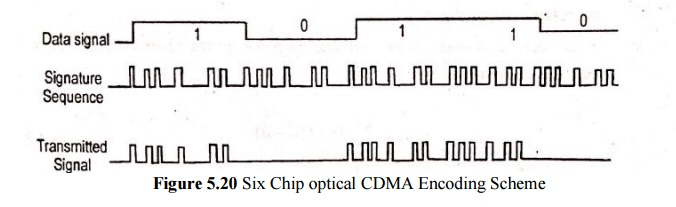# Optical CDMA

The simplest configuration,CDMA achieves multiple access by assigning a unique code to each user.

Optical CDMA

The simplest configuration,CDMA achieves multiple access by assigning a unique code to each user.

To communicate with another node, user imprint their agreed upon code onto the data. The receiver can then decode the bit stream by locking onto the code sequence.

The principle of optical CDMA is based on spread-spectrum techniques.

The concept is to spread the energy of the optical signal over a frequency band that is much wider than the minimum bandwidth required to send the information.

Spreading is done by a code that is independent of the signal itself.

On optical encoder is used to map each bit of information into the high-rate (longer-code-length) optical sequence.

The symbols is the spreading code are called chips.

The energy density of the transmitted waveform is distributed more or less uniformly over the entire spread-spectrum bandwidth.

The set of optical sequences becomes a set of unique ‘address codes or signature sequences’ the individual network users.The signature sequence contains six chips. When the data signal contains 1 data bit, the six-chip sequence is transmitted, no chips are sent for a 0 data bit.

Time-domain optical CDMA allows a number of users to access a network simultaneously, through the use of a common wavelength.

Both asynchronous and synchronous optical CDMA techniques. In synchronous accessing schemes follow rigorous transmission schdules, the produce more successful transmission (higher throughputs) than asynchronous methods where network access is random and collisions between users can occurs.

An optical CDMA network is based on the use of a coded sequence of pulses.

The setup consists of N transmitter and receiver pairs interconnected in a starTo send information from node j to node k, the address code for node k is impressed upon the data by the encoder at node j.

At the destination, the receiver differentiates between codes by means of correlation detection.If the received signal arrives at the correct destination, then s(n)=f(n).

Equation (5.57) represents an autocorrelation function, if s(n) not equal to  f(n) the equation (5.57) represents a cross-correlation function.

For a receiver to be able to distinguish the proper address correctly, it is necessary to maximize the autocorrelation function and minimize the cross-correlation function.

Prime-sequence codes and optical orthogonal codes (OOCs) are the commonly used spreading sequences in optical CDMA systems.

An OOC systems the number of simultaneous user an is bounded byStudy Material, Lecturing Notes, Assignment, Reference, Wiki description explanation, brief detail
Optical Communication and Networking : Optical Networks : Optical CDMA |